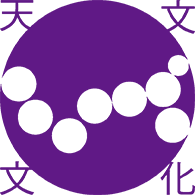>1:(B,\$B@6(B,\$B\$^\$D\$&\$i(B,\$B\$-\$h\$7(B,\$B2#;3(B,\$B7CM}(B,\$B\$h\$3\$d\$^(B,\$B\$(\$j(B,\$BD;5o(B,\$BN4(B,\$B\$H\$j\$\$(B,\$B\$?\$+\$7(B,\$BDMK\C#Li(B,\$B\$D\$+\$b\$H(B,\$B\$?\$D\$d(B,\$BJFED(B,\$BC#O:(B,\$B\$h\$M\$@(B,\$B\$?\$D\$m\$&(B" >

Establishment of cultural astronomy:
Integration of arts and sciences on cultural heritage, astronomical phenomena, and mathematical understanding.

## \$B:G?7>pJs(B

\$B \$B8=:_\$N%W%m%0%i%`Ey>\:Y\$O!\$(B\$B\$3\$A\$i(B[pdf]\$B!%(B
\$B2a5n\$N%W%m%0%i%`\$J\$I\$O!\$(B\$B8&5f2q%Z!<%8(B\$B\$X!%(B

\$BKhF|J82=%;%s%?!<(B\$B\$N%Z!<%8\$K!\$8&5fJ,C4 \$B3X1`9-Js;o!V(BFlow\$B!W(B85\$B9f(B(2019/8)\$B\$K!\$8&5fJ,C4R2p\$5\$l\$^\$7\$?!%(B
\$B3X1`9-Js;o!V(BFlow\$B!W(B84\$B9f(B(2019/4)\$B\$K!\$8&5fJ,C4>1:\$N3hF0\$,>R2p\$5\$l\$^\$7\$?!%(B

### \$BE7J8J82=8&5f2q(B2007\$BG/\$h\$j3+:E\$7\$F\$\$\$k8&5f2q\$N5-O?!%

### \$B%W%m%8%'%/%H(B\$B!VE7J8J82=3X\$NAO@_!W\$r%?%\$%H%k\$K\$7\$?2J3X8&5fHqJd=u6b\$,(B2019\$BG/EY!\$:NBr\$5\$l\$^\$7\$?!%(B

### \$B99?75-O?(B

\$B%m%4%^!<%/\$r\$D\$/\$j\$^\$7\$?!J(B2019\$BG/(B9\$B7n!K(B
\$B%[!<%`%Z!<%8\$r3+@_\$7\$^\$7\$?!J(B2019\$BG/(B7\$B7n!K(B
\$B2J8&Hq(B \$BD)@oE*8&5f!JK(2j!K!VE7J8J82=3X\$NAO@_!W\$,:NBr\$5\$l\$^\$7\$?!J(B2019\$BG/(B7\$B7n!K(B